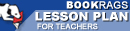Lesson Plans

# Journey Through Genius: The Great Theorems of Mathematics Quiz | Eight Week Quiz F

This set of Lesson Plans consists of approximately 142 pages of tests, essay questions, lessons, and other teaching materials.
 View a FREE sampleName: _________________________ Period: ___________________

This quiz consists of 5 multiple choice and 5 short answer questions through A Sampler of Euler's Number Theory.

## Multiple Choice Questions

1. Who was the first of ancient philosophers to consider why geometric properties existed?
(a) Aristotle.
(b) Pythagoras.
(c) Thales.
(d) Hippocrates.

2. Dunham showed that Heron's proof could also be used as which of the following?
(a) A proof of Archimedes' number theory.
(b) A proof of Euclid's number theory
(c) A proof of the Pythagorean Theorem.
(d) A proof of Hippocrates' squared areas.

3. What did Dunham consider extraordinary about the Elements?
(a) How Hippocrates ordered the book.
(b) How geometric proofs were presented.
(c) The content was totally unique.
(d) The content was not based on previous authors' work.

4. What did British scholars accuse Leibniz of?
(a) Stealing Newton's Binomial theorem.
(b) Publishing Newton's work without his approval.
(c) Plagiarizing Newton's calculus method.
(d) Conspiring the death of Newton.

5. Which of the following is true in modern math about twin primes?
(a) We don't know if they are finite or infinite.
(b) They are infinite.
(c) They are not considered whole numbers.
(d) Their sum is always another prime number.

## Short Answer Questions

1. Which of the following was true about Cardano, according to Dunham?

2. Who eventually solved the sum of the successive squared denominator series?

3. What was Euclid's definition of a prime number?

4. Which of the following was NOT one of the basic definitions in Elements?

5. Which name does NOT belong?

(see the answer key)

 This section contains 272 words (approx. 1 page at 300 words per page) View a FREE sampleCopyrightsJourney Through Genius: The Great Theorems of Mathematics from BookRags. (c)2019 BookRags, Inc. All rights reserved.
Follow Us on Facebook